## Site Settings

##### Currency settings
Products
RF
EMI
Resources
Product Documentation
Handling & Processing
Models & Layout Tools
Education
General
Video Library
Cx Family Common Mode Chokes
0402CT Low Profile Chip Inductors
XAL7050 High-inductance Shielded Power Inductors
XGL4020 Ultra-low DCR Power Inductors
Quality
Quality Certification
Material Certification
Safety Certification
Reliability
Handling / Processing
About
About
Careers
Locations
Resources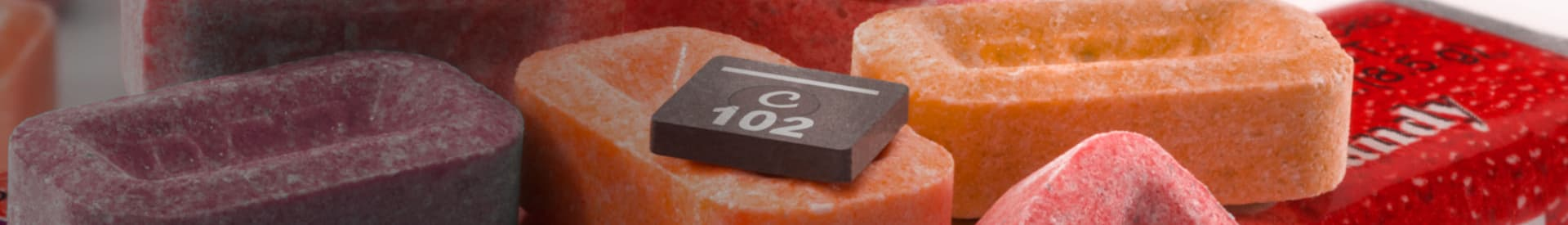# How to use our models in Keysight GENESYS

Note: Even the most current version of Genesys may not contain up-to-date libraries of our inductor models and S-parameters. The Coilcraft SPICE models page provides the most current and accurate versions of our models on our web site.

### S-parameters

S-parameters are convenient to use, but the frequency resolution may not be adequate for narrowband simulations. Yield analysis is not possible with S-parameters. If the frequency resolution is inadequate or if you wish to perform a yield analysis, use our lumped element (SPICE) models (see below).

Our S-parameters are provided in a single compressed "zipped" file. The file must be "unzipped" after downloading to obtain the separate inductor value S-parameter files.

1. Click on the "Linear" element menu.
2. Click on the "Two Port (2)" icon, and select "Two Port (Generic)".
3. Place the generic two-port element onto your schematic.
4. Double-click on the element.
5. Click the "Browse" button.
6. Browse to the folder where the unzipped files are located and select the desired S-parameter file.
7. Click "OK".
8. Add a ground to the ground port.

To view the S-parameter characteristics only of this part, add an input port to the "1" port, and an output port to the "2" port.

To connect the S-parameter element into your circuit, wire the input and output ports to other components in your schematic.

### Lumped element (SPICE) models

To model our rf chip inductors, each individual lumped element (R2, RVAR1, L, C and R1) for the complete model must be placed into the schematic. An example chip inductor schematic is shown in Figure 1.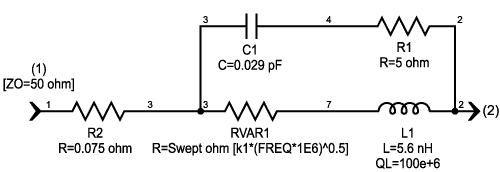Figure 1. Example schematic for a Coilcraft chip inductor

To model our power inductors, each individual lumped element (R2, RVAR1, LVAR, C, R1, RVAR2) for the complete model must be placed into the schematic. An example of a power inductor schematic is shown in Figure 2.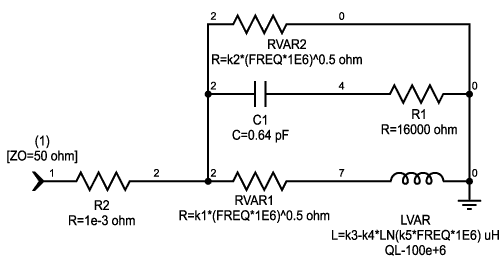Figure 2. Example schematic for a Coilcraft power inductor

After all the lumped elements are placed into the schematic, change the value for each element to match the value in the model table for the specific inductor. If you plan to model different Coilcraft inductors in the same schematic, be sure to rename the individual lumped elements and variables, then update the values from the model table for each inductor.

Important! All examples below use MHz as the project frequency units. Eagleware Genesys requires conversion of frequency units used in frequency-dependent equations.

For example: For frequency units in:
kHz, multiply "FREQ" by 1e3
Hz, multiply "FREQ" by 1e6
Hz, multiply "FREQ" by 1e9.

The ideal inductor lumped element in Eagleware Genesys has an optional Q value. Make sure that this Q value is set to a very high number (such as 100e6).

### For a frequency-dependent resistance (RVAR1, RVAR2)

Note: This example uses RVAR1 ( = k1 * sqrt (Frequency in MHz)).
1. Place an ideal resistor on the schematic.
2. Double click on the resistor to edit the value.
3. Rename it to identify it as a variable resistor (e.g. "Rvar1").
4. For the resistance value, enter the equation: k1 * (FREQ * 1E6)^0.5.
5. Replace k1 with the value from the model table.

### For a frequency-dependent inductance (LVAR)

Note: This element is used in some of our power inductor models.
1. Place an ideal inductor on the schematic.
2. Double click on the inductor to edit the value.
3. Rename it to identify it as a variable inductor (e.g. "Lvar"). Note: The "LN" function in the following equation is the natural logarithm, base e, not base 10.
4. For the inductance value, enter the equation: k3-k4*LN(k5*FREQ*1E6).
5. Replace k3, k4, and k5 with the values from the model table.
6. For the Q value, enter a very large number, such as 100e6.

### Graphing specific model inductance and Q results

Important! Our models represent de-embedded measurements in which fixture parasitic reactances have been removed. Fixture (or circuit board) parasitic reactances raise the effective impedance (and the effective inductance), lower the self-resonant frequency (SRF), and shift the Q curve. For the most accurate model of our inductors in your specific circuit environment, you must include your circuit board model in the simulation.

### Inductance (for versions 2004 and earlier)

To view the effective series inductance of the model in a graph:

Note: This example multiplies the inductance result by 1e9 to convert to nH units. You must modify this factor to adjust it to the applicable inductance units if different from nH (e.g. change 1e9 to 1e6 for uH).
1. Right-click on "Outputs" in the Workspace window tree.
2. Click on "Add rectangular Graph."
3. Enter a name for your graph.
4. In the "Default Simulation/Data or Equations", select your simulation of the model schematic.
5. Click on the "Equation Wizard." button.
6. Select "Insert Measurement."
7. Select the appropriate simulation of your model schematic.
8. Select "ZINi" as the measurement, and "Im [ ]" as the display format.
9. Select your model input port (e.g. ZIN1) as your "Specific Item."
10. Click "Finish."
11. Click in the "Equation" edit window at the end of your equation to continue.
12. Type " *1e9 / (2 * PI * FREQ * 1e6)" to complete the inductance calculation.
13. Click "Finish", then "OK."
14. Click "OK" to complete the graph.

### Inductance (for versions 2004 and earlier)

Measuring inductance in Genesys 2005 is different than in previous releases. Start by creating two new variables in the "Equations" window:

```            inputZ = im(zin(Linear1_Sch1_Data.S[1,1],Linear1_Sch1_Data.ZPORT))
L_model = (inputZ./(2*PI*Linear1_Sch1_Data.F))*1e6```

Once these variables are created, they can be accessed in rectangular graphs or as outputs in the "data" section of the simulation. To see a tabular output, open the Data section of the simulation and right click in the variable section and select "add new variable".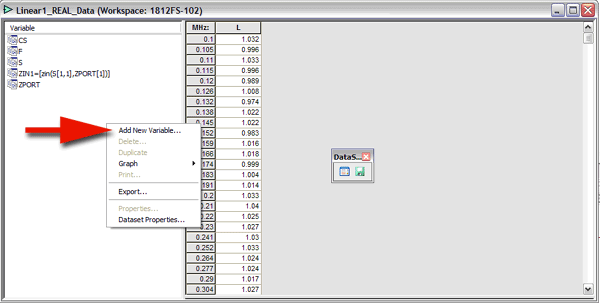Enter the variable "L_model" into the formula prompt as "L" or "Inductance". The inductance is output in the data section.

To create a rectangular graph, select "Equations" in the Dataset section. Enter "L_model" into the measurement section of the graph properties.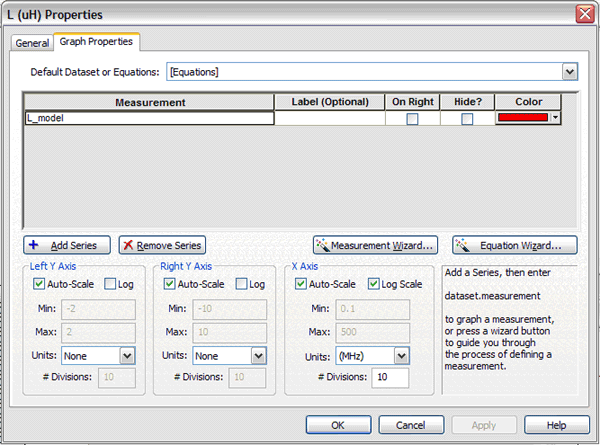### Quality factor (Q)

Q values and curves in our data sheets are typically based on measurements using an impedance analyzer in a 50 Ohm environment, giving a 1-port (reflection) measurement result. If Q calculations are to be compared with data sheet values and curves, they should be based on a simulation with one port of the inductor model connected to ground (as shown in Figure 2).

If you are interested in the Q of the inductor in a 2-port series configuration, the additional 50 Ohms impedance of the second port results in a lower simulated Q value than the 1-port configuration. This result is logical considering that the additional 50 Ohms applies to the "Re [Z]" in the denominator of the Q calculation equation.

### Q = Im [Z] / Re [Z]

To view the Q of the model in a graph:

1. Right-click on "Outputs" in the Workspace window tree.
2. Click on "Add rectangular Graph" and enter a name for your graph.
3. In the "Default Simulation/Data or Equations", select your simulation of the model schematic.
4. Click on the "Equation Wizard." button.
5. Select "Insert Measurement."
6. Select the appropriate simulation of your model schematic.
7. Select "ZINi" as the measurement, and "Im [ ]" as the display format.
8. Select your model input port (e.g. ZIN1) as your "Specific Item."
9. Click "Finish."
10. Click in the "Equation" edit window at the end of your equation to continue.
11. Type "/" (to divide by the next measurement).
12. Click on the "Equation Wizard." button.
13. Select "Insert Measurement."
14. Select the appropriate simulation of your model schematic.
15. Select "ZINi" as the measurement, and "Re [ ]" as the display format.
16. Select your model input port (e.g. ZIN1) as your "Specific Item."
17. Click "Finish", then "OK."